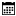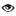#### Sample Programs

Murugan Andezuthu Dharmaratnam |02 March 2021 |11051

Reading a number from the keyboard is one of the programs which is required while coding in 8086 assembly language. In 8086 reading a number from the keyboard is not very simple like how you could read it in C or C . Here you have to read character by character and convert the characters to a number. The below code can read any number from 0 to 65536 which is a word or two bytes. You press enter when you have entered the number. This is what we are doing in the code. Let's take an example where I have to input a number 255

```We have a variable number that will store the output

1. set value of the number to 0
2. First The User Enters 2
3. check if enter key is pressed. If pressed go to number 6
4. multiply the variable number by 10 will give number = 0 because the value of a variable number is 0, zero multiplied by any number is 0
5. add 2 to a variable number

Then you go to step 1 and repeat it till enter key is pressed. Let me tell you what happens when the user press 5.
in step 4. number = number * 10 = 20 because 2 was entered earlier. add 5 to variable number. then the number will be 25
go to step 1
user press number 5 again in step 4 = number = number * 10 = 250 because 25 was already there in number add 5 again to the number and the value becomes 255

6. Here you can write code to do whatever after you have the number in a variable number

```

## 8086 Program to read a number using keyboard

```.model tiny
.code
org 100h

main proc near

loop_number_main:
mov ah,09h  ; dos function 09h to print a string
mov dx,offset messageinputnumber    ; memory location of message
int 21h     ; dos interrupt 21h

mov number,0    ; set initial value of number to 0

mov ah,01h  ; dos function to read a character with echo from keyboard
; result (character entered) is stored in al
int 21h     ; dos interrupt 21h

cmp al,0dh  ; check if enter key is pressed

cmp al,30h  ; check if input character is less then 0,
; here we are validating user input to check if entered character is betwen 0 and 9

cmp al,39h  ; check if input character is great then 9

sub al,30h  ; subtract 30h character code of character 0 from input to get numeric value
mov ah,00
mov bx,ax   ; temporarily store value of read character in bx

mov ax,number   ; copy number to ax
mul numberplace ; we multipley number with 10.
; first time the number is 0 so multiplication with 10 it will remain same

mov number,ax

invalidcharacter:
mov ah,09h  ; dos function 09h to print a string
mov dx,offset messageinvalidcharacter   ; memoery location of message
int 21h     ; dos interrupt 21h

jmp loop_number_main

numbercomplete:
; When the user press enter the control will transfer to this location
; decimal value of input numeric charaters  is stored in variable number

mov ax,number ; this line can be removed. I am addint this only so that
; you can check the value of number in ax register in emu8086

; Here you can write code to display the number
; or use entered number as an input for other program

mov ah,4ch      ; dos function 4ch to terminate and return to dos
mov al,00
int 21h         ; dos interrupt 21h

endp
numberplace db 10
number dw 0
messageinvalidcharacter db 0ah,0dh,"Invalid Character\$"
messageinputnumber db 0ah,0dh,"Please Input a number \$"
end main

```